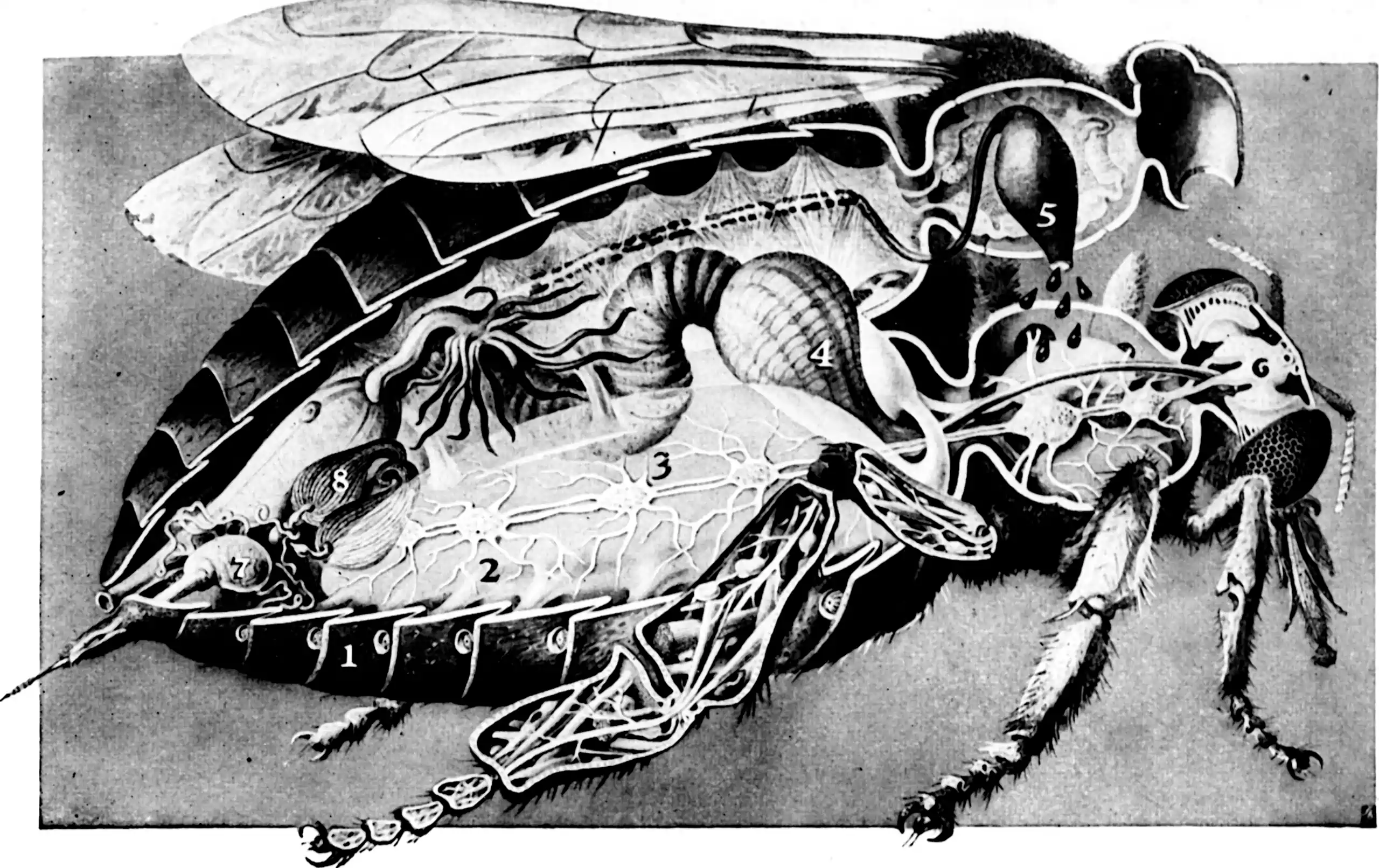# Bayes linear regression and basis-functions in Gaussian process regression

## a.k.a Fixed Rank Kriging, weight space GPsNo, these are officers. You want low rank Gaussian processes.

Another way of cunningly chopping up the work of fitting a Gaussian process is to represent the process as a random function comprising basis functions $$\phi=\left(\phi_{1}, \ldots, \phi_{\ell}\right)$$ with the Gaussian random weight vector $$w$$ so that $f^{(w)}(\cdot)=\sum_{i=1}^{\ell} w_{i} \phi_{i}(\cdot) \quad \boldsymbol{w} \sim \mathcal{N}\left(\mathbf{0}, \boldsymbol{\Sigma}_{\boldsymbol{w}}\right).$ $$f^{(w)}$$ is a random function satisfying $$\boldsymbol{f}^{(\boldsymbol{w})} \sim \mathcal{N}\left(\mathbf{0}, \boldsymbol{\Phi}_{n} \boldsymbol{\Sigma}_{\boldsymbol{w}} \boldsymbol{\Phi}^{\top}\right)$$, where $$\boldsymbol{\Phi}_{n}=\boldsymbol{\phi}(\mathbf{X})$$ is a $$|\mathbf{X}| \times \ell$$ matrix of features. This is referred to as a weight space approach in ML.

TODO: I just assumed centred weights here, but that is crazy. Update to relax that assumption.

We might imagine this representation would be exact if we had countably many basis functions, and under sane conditions it is. We would like to know, further, that we can find a basis such that we need not too many basis functions to represent the process. Looking at the Karhunen-Loève theorem theorem we might imagine that this can sometimes work out fine, and indeed it does, sometimes.

This is a classic; see Chapter 3 of Bishop (2006) is classic and nicely clear. Cressie and Wikle (2011) targets for the spatiotemporal context.

Hijinks ensue when selecting the basis functions. If we were to treat the natural Hilbert space here seriously we could consider identifying the bases as eigenfunctions of the kernel. This is not generally easy. We tend to use either global bases such as Fourier bases or more generally Karhunen-Loéve bases, or construct local bases of limited overlap (usually piecewise polynomials AFAICT).

## Fourier features

When the Fourier basis is natural for the problem we are in a pretty good situation. We can use the Wiener Khintchine relations to analyse and simulate the process.

## Random Fourier features

The random Fourier features method constructs a Monte Carlo estimate to a stationary kernel by representing the inner product in terms of $$\ell$$ complex exponential basis functions $$\phi_{j}(\boldsymbol{x})=\ell^{-1 / 2} \exp \left(i \boldsymbol{\omega}_{j}^{\top} \boldsymbol{x}\right)$$ with frequency parameters $$\boldsymbol{\omega}_{j}$$ sampled proportionally to the spectral density $$\rho\left(\boldsymbol{\omega}_{j}\right).$$

This sometimes has a favourable error rate .

## K-L basis

We recall from the Karhunen-Loéve notebook that the mean-square-optimal $$f^{(w)}$$ for approximating a Gaussian process $$f$$ is found by truncating the Karhunen-Loéve expansion $f(\cdot)=\sum_{i=1}^{\infty} w_{i} \phi_{i}(\cdot) \quad w_{i} \sim \mathcal{N}\left(0, \lambda_{i}\right)$ where $$\phi_{i}$$ and $$\lambda_{i}$$ are, respectively, the $$i$$-th (orthogonal) eigenfunction and eigenvalue of the covariance operator $$\psi \mapsto \int_{\mathcal{X}} \psi(\boldsymbol{x}) k(\boldsymbol{x}, \cdot) \mathrm{d} \boldsymbol{x}$$, written in decreasing order of $$\lambda_{i}$$. What is the orthogonal basis $$\{\phi_{i}\}_i$$ though? That depends on the problem and can be a lot of work to calculate.

In the case that our field is stationary on a “nice” domain, though, this can easy — we simply have the Fourier features as the natural basis.## Compactly-supported basis functions

As seen in GPs as SDEs and FEMs .

## “Decoupled” bases

Cheng and Boots (2017);Salimbeni et al. (2018);Shi, Titsias, and Mnih (2020);Wilson et al. (2020).

### No comments yet. Why not leave one?

GitHub-flavored Markdown & a sane subset of HTML is supported.